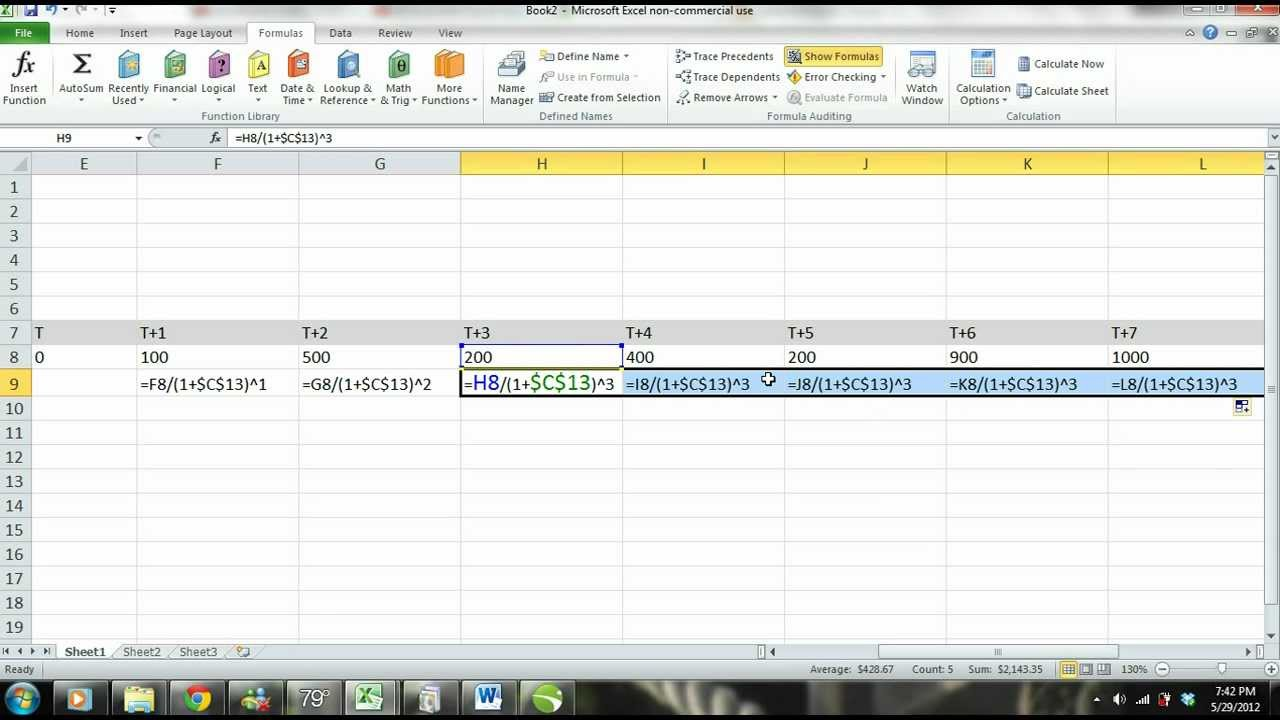## Excel Tips and Tricks: Present Value of Multiple Uneven Cash Flows – Excel Tutorial

http://www.excelfornoob.com
http://www.subjectmoney.com

In this Microsoft Excel 2010 tutorial we explain the concept of present value and show you how to find the present value of multiple cash flows using MS Excel 2010. In this lesson w show you how to solve for the PV of multiple cash flows by entering the PV formula manually and then we also show you how to solve the PV of multiple uneven cash flows using the NPV formula